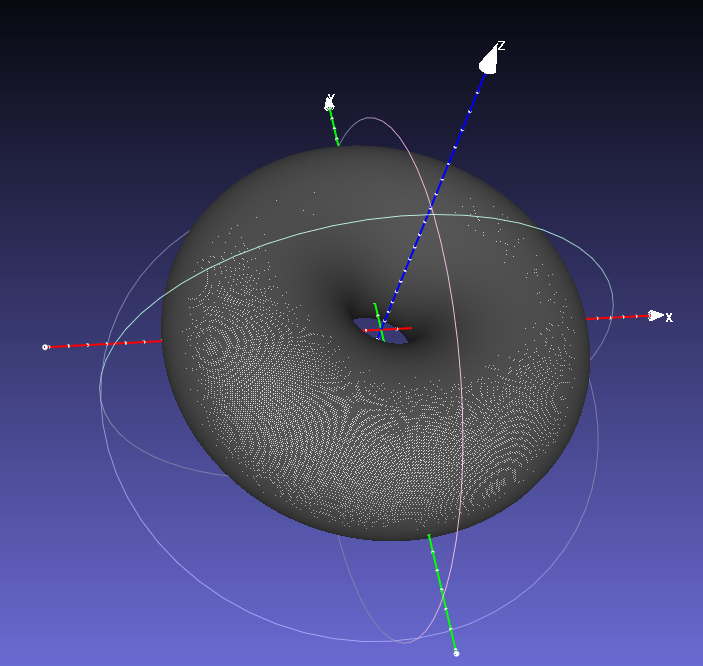Defining A Wall From A 2D Polygon¶

When studying candidate reactor designs for future machines, detailed engineering meshes are not always available. Sometimes all we have is a simple 2D outline representing a candidate wall design.

In this example we make a 3D mesh from a simple outline. The wall mesh is intended to wrap the plasma emission function from the previous radiation function example. The utility function axisymmetric_mesh_from_polygon() is useful for creating a 3D mesh from a 2D polygon.

import numpy as np
from raysect.core import Point2D
from raysect.primitive import export_obj

from cherab.tools.primitives import axisymmetric_mesh_from_polygon

PLASMA_AXIS = Point2D(1.5, 0)

CENTRE_PEAK_WIDTH = 0.05
RING_WIDTH = 0.025

# distance of wall from LCFS
WALL_LCFS_OFFSET = 0.1

CYLINDER_HEIGHT = (LCFS_RADIUS + WALL_LCFS_OFFSET) * 2

##########################################
# make toroidal wall wrapping the plasma #

# number of poloidal wall elements
num = 250
d_angle = (2*np.pi) / num

wall_polygon = np.zeros((num, 2))
for i in range(num):
pr = PLASMA_AXIS.x + WALL_RADIUS * np.sin(d_angle * i)
pz = PLASMA_AXIS.y + WALL_RADIUS * np.cos(d_angle * i)
wall_polygon[i, :] = pr, pz

# Note - its important for this application that the resulting mesh triangles
# are facing inwards. This will be determined by the polygon winding direction.
# Positive winding angles produce outward facing meshes so we need to flip
# the polygon to achieve an inward facing mesh.
wall_polygon = wall_polygon[::-1]

# create a 3D mesh from the 2D polygon outline using symmetry
wall_mesh = axisymmetric_mesh_from_polygon(wall_polygon)

# write out the mesh to the OBJ triangular mesh format for visualisation in external tools
# (e.g. Meshlab, Blender, etc)
export_obj(wall_mesh, 'toroidal_wall.obj')Caption: The resulting toroidal mesh visualised in Meshlab.